# Residential Air Flowmeter

Have you ever wanted to measure the speed of air coming out of your vents at home? Well then we have the perfect project for you.

BeginnerFull instructions provided4,983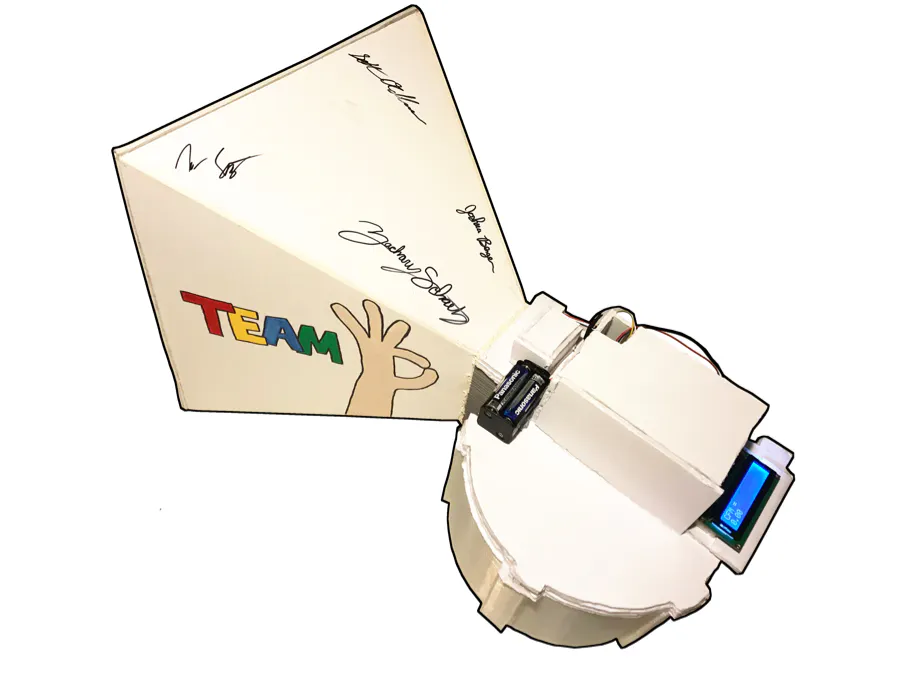## Things used in this project

### Hardware componentsArduino UNO & Genuino UNO
×1Jumper wires (generic) 25 Wires in total
×25×1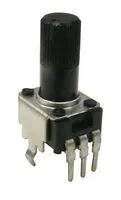Rotary Potentiometer, 10 kohm
×1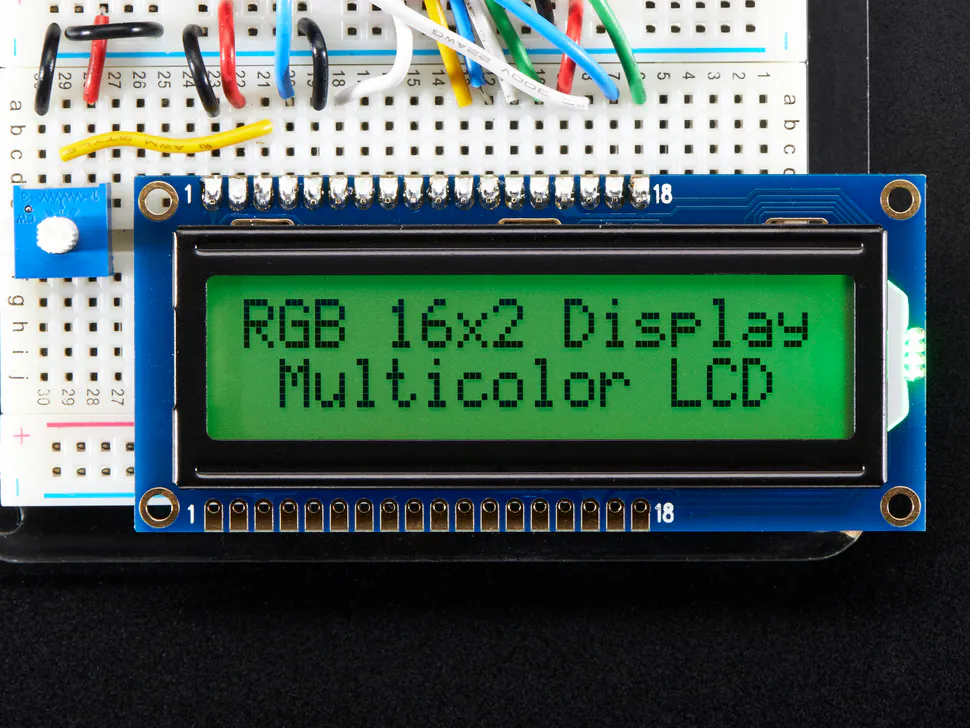Adafruit RGB Backlight LCD - 16x2
×1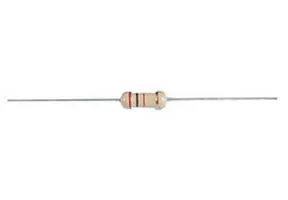Through Hole Resistor, 220 kohm
×1
 Diymall Voltage Sensor Dc0-25v
×1
×1
×1

### Hand tools and fabrication machinesSoldering iron (generic)

## Schematics

### Wiring Diagram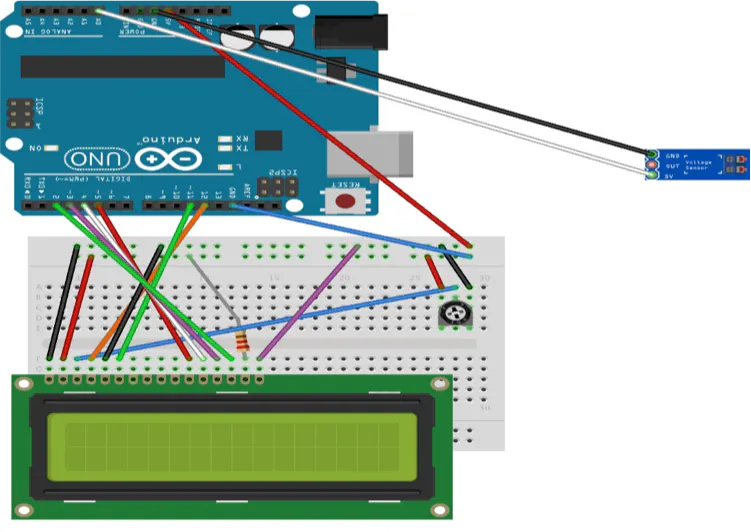## Code

### Flowmeter Code

C/C++
```1.	#include <LiquidCrystal.h>
2.
3.	LiquidCrystal lcd(12, 11, 5, 4, 3, 2);
4.	// These are the pins that will be used
5.
6.	 // Below are the values of the resistors in the sensor
7.	float R1=30000;
8.
9.	float R2=7500;
10.
11.	void setup()
12.	{
13.
14.	Serial.begin (9600);
15.	lcd.begin(16, 2);
16.	 // This allows the LCD screen to be used
17.
18.
19.	}
20.
21.	void loop()
22.	// Beginning of the loop
23.	{
24.
26.	 // Reads  the sensor value
27.
28.	float voltage = ((5*sensorValue*(R1+R2))/(1024*R2));
29.	 // Equation for the real voltage
30.
31.
32.	//*********************************************************//
33.	// The equation for CFM is a piecewise function of voltage,
34.	// so if statements were used to divide the functions into
35.	// their respective regions below
36.	//*********************************************************//
37.
38.
39.	if (voltage > 1)
40.	 // First region of the function for large CFM
41.	{
42.
43.	float cfm = (1.1409*(voltage))*((voltage))+44.258*(voltage);
44.
45.	Serial.println(cfm);
46.	 // Displays CFM
47.	lcd.print("CFM = ");
48.	lcd.setCursor(0, 1);
49.	lcd.print(cfm);
50.	delay(1000);
51.	 // 1000 ms delay
52.	lcd.clear();
53.	 // Clears for repeat
54.	delay(1000);
55.
56.	}
57.
58.	else if (.01 < voltage < 1)
59.	 // Second region for middle to low CFM
60.	{
61.
62.	float cfm = (40*(voltage)+20);
63.
64.	Serial.println(cfm);
65.	// Displays CFM
66.	lcd.print("CFM = ");
67.	lcd.setCursor(0, 1);
68.	lcd.print(cfm);
69.	delay(1000);
70.	 // 1000 ms delay
71.	lcd.clear();
72.	 // Clears for repeat
73.	delay(1000);
74.
75.	}
76.
77.	else
78.	 // If fan is not spinning, the CFM will be zero
79.	{
80.	  float cfm = 0;
81.
82.	  Serial.println(cfm);
83.	lcd.print("CFM = ");
84.	lcd.setCursor(0, 1);
85.	lcd.print(cfm);
86.	delay(1000);
87.	lcd.clear();
88.	delay(1000);
89.	}
90.
91.
92.	}
```

### Code for Voltage Sensor

C/C++
This is the code to read the voltage if you want to calibrate it yourself
``` // Below are the values of the resistors in the sensor
float R1=30000;
float R2=7500;

void setup() {

Serial.begin (9600);

}

void loop() {

// Equation for the real voltage
float voltage = ((5*sensorValue*(R1+R2))/(1024*R2));

// Prints voltage on computer
Serial.printIn(voltage);
delay(1000);
}
```

### Voltage Sensor Code

C/C++
This is the code you use to calibrate the Flowmeter yourself.
```#include <LiquidCrystal.h>

LiquidCrystal lcd(12, 11, 5, 4, 3, 2);                                    // These are the pins that will be used

// Below are the values of the resistors in the sensor
float R1=30000;
float R2=7500;

void setup()
{

// This allows the LCD screen to be used
Serial.begin (9600);
lcd.begin(16, 2);

}

void loop()
{

// Equation for the real voltage
float voltage = ((5*sensorValue*(R1+R2))/(1024*R2));

// Shows voltage on the LCD screen
Serial.println(voltage);
lcd.print("V = ");
lcd.setCursor(0, 1);
lcd.print(voltage);
delay(1000);
lcd.clear();
delay(1000);

}
```

## Credits

### Ardunicko

0 projects • 1 follower